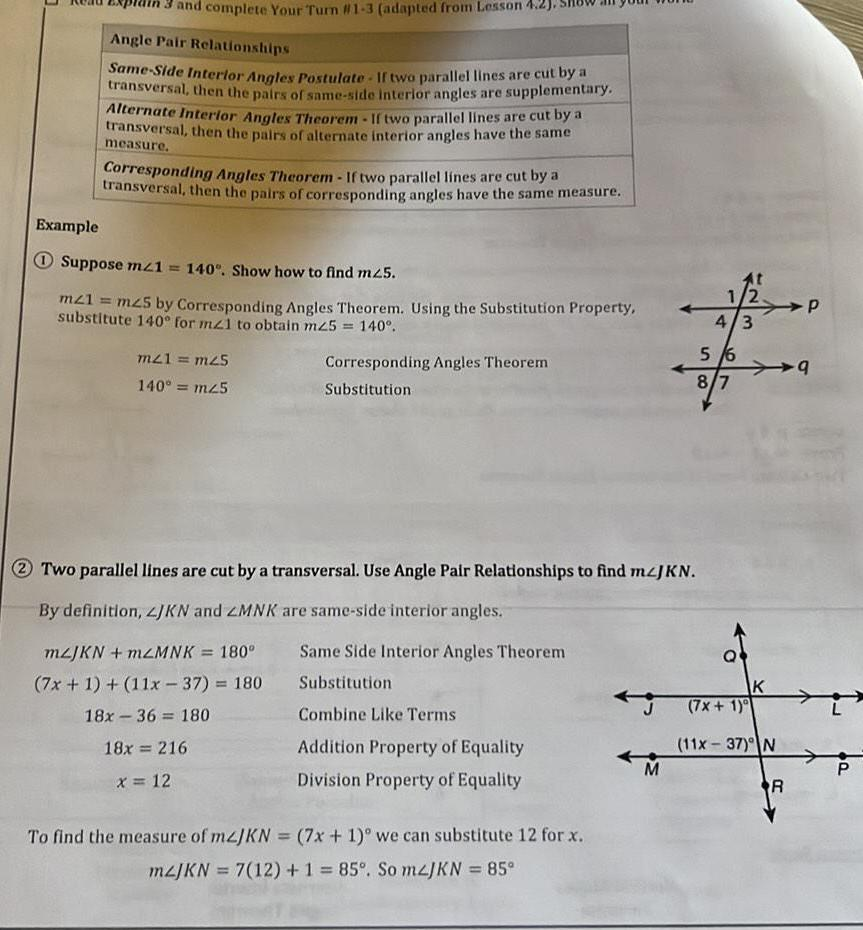Question:

# 13 and complete Your Turn 1 3 adapted from Lesson 4 2 Angle

Last updated: 11/21/202313 and complete Your Turn 1 3 adapted from Lesson 4 2 Angle Pair Relationships Same Side Interior Angles Postulate If two parallel lines are cut by a transversal then the pairs of same side interior angles are supplementary Alternate Interior Angles Theorem If two parallel lines are cut by a transversal then the pairs of alternate interior angles have the same measure Corresponding Angles Theorem If two parallel lines are cut by a transversal then the pairs of corresponding angles have the same measure Example Suppose m 1 140 Show how to find m25 m21 mz5 by Corresponding Angles Theorem Using the Substitution Property substitute 140 for mz1 to obtain m25 140 mz1 m25 140 mz5 Corresponding Angles Theorem Substitution To find the measure of mzJKN 2 Two parallel lines are cut by a transversal Use Angle Pair Relationships to find mzJKN By definition JKN and ZMNK are same side interior angles mzJKN mzMNK 180 7x 1 11x37 180 18x 36 180 18x 216 x 12 Same Side Interior Angles Theorem Substitution Combine Like Terms Addition Property of Equality Division Property of Equality 7x 1 we can substitute 12 for x mzJKN 7 12 1 85 So m2JKN 85 M 4 3 5 6 8 7 K 7x 1 11x 37 N R P 9 L P